# Determination of the number of shots for Grover’s search algorithm

## Abstract

This paper focuses on Grover’s quantum search algorithm, which is of paramount importance as a masterpiece of Quantum Computing software. Given the inherent probabilistic nature of quantum computers, quantum programs based on Grover’s algorithm need to be run a number of times in order to generate a histogram of candidate values for solutions, which are then checked to identify the valid ones. In this paper, the distribution of the required number of shots to find all or a fraction of all the solutions to the Grover’s search problem is studied. Firstly, considering the similarity of the probability problem with the well-known coupon collector’s problem, two formulae are obtained from asymptotic results on the distribution of the required number of shots, as the number of problem solutions grows. These expressions allow to compute the number of shots required to ensure that, with probability p, all or a fraction of all the solutions are found. Secondly, the probability mass function of the required number of shots is derived, which serves as a benchmark to assess the validity of the asymptotic approximations derived previously. A comparison between the two approaches is presented and, as a result, a rule of thumb to decide under which circumstances employ one or the other is proposed.

## 1 Introduction

Grover’s algorithm  is one of the most important and applied algorithms in quantum computing. The original algorithm has been demonstrated to be optimal for the search problem . It has been extended to solve problems with multiple solutions  and to improve the probability of finding them . It is also the base for the more general amplitude amplification and amplitude estimation algorithms , which are fundamental parts of other quantum algorithms. Grover’s algorithm has been successfully applied to many problem domains such as image recognition , cryptography , global optimization , string matching , quantum chemistry , genetic algorithm , fuzzy systems , Boolean satisfiability problems , and machine learning [19, 20], to mention a few.

Grover’s algorithm relies on the existence of an oracle function that is able to identify a solution to a search problem of size N. By exploiting the quantum effect of superposition, it can find an item in an unordered dataset with only $$\Theta (\sqrt {N} )$$ evaluations of the oracle, $$\Theta (\sqrt {N/M} )$$ when the search problem has M solutions. This is an improvement over the $$\Theta (N)$$ evaluations required by classical algorithms. It comes with the drawback, however, that it is a probabilistic algorithm and the value it outputs is, therefore, not always a solution to the problem. This probability depends on the number of Grover iterations i (i.e., one oracle evaluation plus an execution of the Grover diffuser)  in the quantum circuit, the size of the space to be explored and the number of solutions :

$$p_{G}=\sin ^{2} \bigl((2\cdot i+1)\cdot \theta _{G} \bigr), \quad \text{being } \theta _{G}= \arcsin \biggl(\sqrt{\frac{M}{N}} \biggr).$$
(1)

Another characteristic of Grover’s algorithm is that the value it outputs may coincide with previously obtained ones (whether solutions or not), since each execution is independent of the previous ones. This behaviour is intrinsic to the probabilistic nature of quantum computing and is compensated by repeatedly re-running the circuit a number of times (henceforth, “shots”) and analysing the histogram of values output by the algorithm. Since, by design, Grover’s algorithm maximises the probabilities of finding a solution, the most repeated values in the histogram will most probably be solutions to the search problem.

In this context, this paper focuses on studying the number of shots required to find, with a given probability, the M solutions or a fraction of them.

This question is relevant because there are scenarios in which you may want to get several solutions in order to compare them against other criteria, and because of the scarcity and high cost of quantum computers, it is necessary to use them efficiently, in a sustainable way.

From a probability point of view, the problem under study bears much similarity with the coupon collector’s one which states: if each pack of bubble gum contains a coupon, and a complete set consists of M different coupons, how many packs of bubble gum does one have to buy to get, with probability p, a complete set? [21, Sect. 8.1 and 8.4]. Possibly, the first results published on the coupon collector’s problem can be found in the classical textbook by Feller , where it is called the waiting time problem and the first moments are derived. Since then, many authors have studied in particular the asymptotic distribution of the number of required packs as the size of the complete set increases, considering a range of variations for the sampling scheme, see for example [23, 24] and . In , the mean and variance of the number of trials required to complete the collection are obtained extending the case of equally likely coupons, allowing the probability of selecting a coupon to vary from one coupon to the other, according to an integral formula. In , the classical coupon collector’s problem is extended to one in which two collectors are simultaneously and independently seeking collections, while, more recently, in , asymptotic results for the variance and the distribution of the required number of trials are obtained under quite general conditions on the probabilities of extracting each coupon, achieving more general results than . The substantial difference between the problem studied in this paper and the one considered by the coupon’s collector is that an execution of Grover’s algorithm outputs one of the M solutions with probability $$p_{G}$$ (see (1)), while the collector always gets a coupon when opening a bubble gum pack.

The average number of shots required to find all solutions is straightforward to obtain and could be considered as a first hint or a rule of thumb about the number of shots required by Grover’s algorithm. However, there is no guarantee that the probability to get all solutions is high enough. To gain knowledge and allow sound decisions to be made, information about the whole distribution, not only its expectation or variance, is needed. In this paper, after computing the expectation and variance for the number of required shots, we take advantage of asymptotic results proved in the classical coupon collector’s problem to derive approximations for the number of shots required to find the M solutions, or a fraction of them, with a given probability. Afterwards, we extend the work by providing an exact formula for the probability mass function corresponding to the number of shots required to find the M solutions to the problem or a fraction of them, depending on the user’s needs. This exact formula (i) provides a benchmark to assess when the approximations can be used without incurring large deviations from the exact values, and (ii) can be used when the number of solutions is small, which would put at risk the validity of the asymptotic approximations. Lastly, a comparison between the approximated and the exact formulae is presented and a rule of thumb about when to use the approximated expressions is suggested.

Without loss of generality, the authors of this paper assume that the number of solutions is known in advance (which is a requirement in order to design a quantum circuit with high probability of outputting them), and do not consider the fact that quantum computing devices are noisy, and therefore the values predicted by the formulas described may not coincide with the results of current real world experiments. Regarding the first assumption,  describes a modification of Grover’s algorithm to account for this, while  proposes the inclusion of quantum counting algorithms as a previous step before running Grover’s algorithm. Regarding the second one, the effects of noise have been studied in quantum computing in general, and noise correction mechanisms have been proposed and successfully applied in real quantum computers . The effect of noise in Grover’s algorithm in particular has also been subject to study in research works such as [28, 29]. In any case, we are at the beginning of the quantum era, and quantum hardware development is still very unreliable. But that will change in the coming years, making experiments converge to the values predicted by this paper.

## 2 Results

Denote the number of solutions of the search problem by M and let $$X_{\scriptscriptstyle A, M}$$ be the shot on which, for the first time, the number of different solutions that have been sampled is $$A + 1$$. We are in particular interested, for a given probability $$p\in [0, 1]$$, in the number s that satisfies:

$$s = \inf \bigl\{ r \in \mathbb{N}: {\mathbb{P}}(X_{A, M} \leq r) \geq p \bigr\} ,$$
(2)

where, for a set S, infS denotes the infimum of S, also called the greatest lower bound of S.

The integer s is the answer to the question “how many shots are required to ensure, with probability p, to have found all M solutions of the problem (or a fraction of them) at least once?”. More generally, we are interested in the whole distribution of values of $$X_{A, M}$$. The integer s in (2) is then a quantile of that distribution, properly defined using the generalized inverse of the distribution function, see [30, p. 39]. The generalized inverse is required since the random variable $$X_{A, M}$$ takes discrete values. Several cases regarding A are particularly relevant for this research:

• $$A = M - 1$$. $$X_{M-1, M}$$ is the shot number in which, for the first time, all different solutions have appeared at least once.

• $$A = 0$$. $$X_{0, M}$$ is the first appearance of a solution.

• $$A = k\cdot M - 1$$, for some $$0< k < 1$$. For example, $$k = 0.5$$ means that the interest is in finding $$M/2$$ solutions.

Please note that the quantity $$A = M -1$$ is introduced because it leads to more compact expressions.

### 2.1 Expectation and variance of $$X_{A, M}$$

The first elements of information about the distribution of $$X_{A, M}$$ that can be easily deduced are its expectation and variance. Indeed, $$X_{A, M}$$ has the same distribution as that of the sum of independent variables

$$G_{p_{G}} + G_{p_{G}(M - 1)/M }+\cdots + G_{p_{G}(M-A)/M },$$
(3)

where each $$G_{p}$$ follows a Geometric distribution with parameter p, which models the number of Bernoulli trials required to get a success, which has probability p. The first Geometric distribution $$G_{p_{G}}$$ represents the number of shots required to get a first solution, the second Geometric variable represents the number of shots required to get another solution different from the previous one (probability of success is $$p_{G}(M - 1)/M$$ now), etc.

Since for a Geometric distribution, $${\mathbb{E}}[G_{p}] = 1 / p$$ and $$\operatorname{Var}(G_{p}) = \frac {1 - p}{p^{2}}$$, the expectation and variance of $$X_{A, M}$$ are deduced from (3), where $$p_{G}$$ is defined in (1):

\begin{aligned}& {\mathbb{E}}[X_{A, M}] = \frac{M}{p_{G}} \sum _{i = M - A} ^{M} \frac {1}{i}, \end{aligned}
(4)
\begin{aligned}& \operatorname{Var}(X_{A, M}) = \frac {M^{2}}{p_{G}^{2}} \sum _{i = M - A} ^{M} \frac {1}{i^{2}} - \frac {M}{p_{G}} \sum_{i = M - A} ^{M} \frac {1}{i}. \end{aligned}
(5)

### 2.2 Approximations to the number of required shots

In this section, an approximation to the distribution of $$X_{A, M}$$ is derived, which provides expressions for the number of required shots. This approximation is adapted from the results in . Two cases are considered: when interested in finding all M solutions and when only a fraction of all the solutions is required.

### Proposition 1

In the case when $$A = M - 1$$, i.e., when interested in finding the M solutions,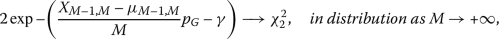(6)

where γ denotes the Euler constant, see [32, Sect. 5.2(ii)] and $$\chi _{2}^{2}$$ denotes a chi-square distribution with 2 degrees of freedom. The expression for $$\mu _{M-1, M} = {\mathbb{E}}[X_{M-1, M}]$$ is given in (4).

As a result, the following approximation for the quantile function holds: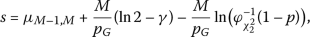(7)

where $$\varphi ^{-1}_{\chi _{2}^{2}}(q)$$ denotes the inverse of the cumulative distribution function of a $$\chi _{2}$$ distribution with two degrees of freedom.

### Proposition 2

In the case when $$A = k\cdot M - 1$$, for some $$0< k<1$$, i.e., when interested in finding a fraction of all solutions,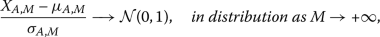(8)

where $$\mathcal{N}(0,1)$$ denotes the Standard Normal distribution, and expressions for $$\mu _{A, M}$$ and $$\sigma _{A, M}^{2}$$ can be found in (4) and (5), respectively.

As a result, the following approximation for the quantile function holds: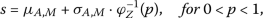(9)

where $$\varphi ^{-1}_{Z}$$ denotes the inverse of the cumulative distribution function of a Standard Normal distribution.

A Python script that implements the approximated cumulative distribution function and the corresponding formulae for s in (7) and (9) can be found on the repository mentioned in the Data availability section. It allows the user to specify A, M, $$p_{G}$$ and choose the probability p to compute the required number of shots s.

On the other hand, in the case when interested in finding all solutions, it is straightforward to see from (7) and (4) that the product $$s\cdot p_{G}$$ only depends on M and p. It is therefore possible to construct a unique statistical table which provides values of the product $$s\cdot p_{G}$$ for different combinations of p (columns) and M (rows). An example of such a table is presented in Table 1.

In the case when the interest is in finding only a fraction of the solutions, the expression (5) of the standard deviation $$\sigma _{A, M}$$ that appears in (9) prevents $$s\cdot p_{G}$$ to eliminate the dependence on $$p_{G}$$ and a different table would be needed for each value of $$p_{G}$$ and for each value of k. For that case, the aforementioned scripts can be used.

### 2.3 Exact probability mass function

The approximation derived in the previous section is based on asymptotic results on the distribution of $$X_{A, M}$$ as M grows to infinity, and is therefore expected to be more accurate as M gets large. It is actually possible to derive the exact probability mass function (pmf) for $$X_{A, M}$$, which allows for more accurate numerical computation of any quantity of interest related to the distribution of $$X_{A, M}$$, in particular its quantiles. This is the main result of this section, presented below. As a benchmark, it is also useful to determine the goodness of the approximations derived in the previous section. This will be illustrated in the discussion section, where the approximations are shown to present very good prediction performance, even for small and moderate values of M.

### Proposition 3

Given $$M > 1$$ and $$0 < A < M$$, we have, for $$s \geq A + 1$$,

$${\mathbb{P}}(X_{A, M} = s) = {\binom{M}{A + 1}} (A + 1)! \sum_{l = A} ^{s-1} { \binom{s-1}{l}} \begin{Bmatrix} l \\ A \end{Bmatrix} \biggl({\frac {p_{G}}{M}} \biggr) ^{l + 1} (1 - p_{G})^{n - 1 - l},$$
(10)

where, for two integers $$k\leq m$$, $\left\{\begin{array}{c}m\\ k\end{array}\right\}$ is the Stirling number of the second kind, that is the number of ways of partitioning a set of m elements into k non-empty subsets, see e.g. , Sect. 1.6, and [32, Sect. 28.6(i), Eq. 28.8.5], which can be computed as follows

$$\begin{Bmatrix} m \\ k \end{Bmatrix} = \sum_{r = 1}^{k} (-1) ^{k - r} \frac { r^{m}}{r! (k-r)!}.$$
(11)

Notice that, in the case when we are interested in the first appearance of a solution, i.e., $$A = 0$$, $$X_{0, M}$$ follows a Geometric distribution of parameter $$p_{G}$$:

$${\mathbb{P}}(X_{0, M} = s) = (1 - p_{G})^{s-1} p_{G}, \quad \textit{for s \geq 1.}$$

Formula (10) can be easily implemented. It allows to compute, for any M, A and $$p_{G}$$, the cumulative ditribution of $$X_{A, M}$$ and therefore compute any related quantity. However, take into account that, as the number of solutions M grows, higher precision and range are needed to compute powers, factorials and binomial coefficients. Moreover, the computation of a quantile s using the exact pmf (10) requires computing all point probabilities up to the first integer for which the cumulative distribution function exceeds p. This may be computationally quite intensive for a large M since both the equation (10) and the expression for the Stirling coefficient of the second kind (11) involve sums. The aforementioned repository also contains Python and C implementations that compute the pmf and cumulative distribution function (cdf) of $$X_{A, M}$$ for given values of $$p_{G}$$, A and M. Both implementations use the GMP library for more precise computations .

For illustration purposes, Fig. 1 and Fig. 2 display, for the case of 100 solutions, the quantile function, i.e., the inverse of the cdf of $$X_{A, M}$$: for a varying probability $$0< p<1$$ in Ox axis, the value of s such that $$P(X_{A, M} \leq s) = p$$ is represented. In Fig. 1, $$A=99$$ and $$M = 100$$, i.e, the interest is in finding all 100 solutions, while in Fig. 2, $$A=49$$ and $$M = 100$$, i.e, we want to get half of the solutions.

For the sake of completeness, the Appendix contains the results and enhancements related to the original coupon collector problem that served as the basis for deriving and proving the propositions in this section.

## 3 Discussion

In this section, the exact quantile and the approximated quantile functions are compared, for different values of A, M and $$p_{G}$$, in order to get insight about from which value of M can we safely switch to the simpler approximated expressions, less computationally demanding, for s.

Firstly, for illustration, Figs. 3 and 4 compare graphically the required number of shots using the approximated expressions (7) and (9), and the exact cdf of (10). The match is excellent in the case when we are interested in obtaining the M solutions and very good in the case when we want to ensure a probability p of finding $$M/2$$ solutions. On the other hand, a more systematic comparison was carried out, assessing the closeness of the exact and approximated expressions using the absolute error for the obtained number of shots. It is expectedly easier to approximate a distribution around its center than at its extremes, so the user defined probability p should be paid particular attention to in the assessment. The absolute error $$\varepsilon _{p, M} = s_{\mathrm{approx}.} - s_{\mathrm{exact}}$$ was computed for a varying p as M increases and for different values of $$p_{G}$$. In Fig. 5, which corresponds to the case when the interest is in getting the M solutions, the distribution of these errors, when p takes values in the interval $$[0.5, 0.9]$$ and $$p_{G}=\{0.7, 0.95, 0.999\}$$ is displayed through a boxplot for each value of M represented in the Ox axis. The more extreme value $$p = 0.99$$ is represented separately through individual points. It is remarkable that, on the one hand, the absolute error is small and stable as M increases. Since the value of required number of shots increases dramatically as M grows, this implies in particular that the relative error drops very fast. As an example, it is lower than 4% for $$M = 10$$ for all $$p\leq 0.99$$ (absolute error not greater than 3). On the other hand, it is also observed that $$\varepsilon _{p, M}$$ is always non negative, which implies that the approximated number of shots always overestimates the exact required number of shots. As a result, the approximated expression provides a conservative estimate of the required number of shots, which is actually associated to a higher probability than the user defined p. Regarding the case when the interest is in getting a fraction ($$k<1$$) of all solutions, a similar study was carried out for several values of k. Figure 6 displays the absolute error as in the previous figure, for the case when $$k = 0.90$$, i.e, when 90% of all solutions are required to appear. The behaviour of the absolute error is similar to the case when interested in observing the M solutions, although in this case, the approximation may underestimate the exact required number of shots. Very similar results were obtained for other values of $$0< k < 1$$, with better approximating performance for smaller values of k. The latter is to be expected since the asymptotic result (8) relies on $$M - A$$ tending to infinity as M grows and, for larger values of k like 90% for example, the quantity $$M - A = 10 \% M$$ grows slowler.

From those results, a reasonable rule of thumb would therefore be: when interested in obtaining the M solution or a fraction of them, the approximations (7) and (9) are adequate if $$M \geq 30$$. This value of $$M \geq 30$$ leads indeed to relative errors lower than 3% even for values of p close to 1.

Notice that even if for large values of M the approximation provides very satisfactory precision and is computationally much faster than the exact formula, the latter is relevant in the case when running a large number of simulations is costly and it is therefore important not to overestimate the number of shots. Morever, the exact pmf is essential to assess the validity of the approximated expressions.

A final remark to conclude the discussion: for the case when we are interested in getting all the solutions, if M is large, it is known, see e.g [35, Sect. 12.3.1] that $$\sum_{i = 1}^{M}\frac {1}{i} = \ln M + \gamma + o(1)$$, where γ is the Euler constant, see [32, Sect. 5.2(ii)]. Consequently, the average number of shots required to get the M solutions can be approximated, if M is large enough, by $$(M\ln M) / p_{G}$$. This is a rule of thumb about how to choose the number of shots of Grover’s algorithm that can be found in the community. However, if one were to choose that number of shots, there is no guarantee that the probability to find the M solutions is satisfactorily high. There is a double reason for that: on the one hand, the expectation of the distribution needs not be located in the right extreme part of the distribution, and on the other hand, $$(M\ln M) / p_{G}$$ is a rough approximation that underestimates the expectation. In fact, using the exact pmf, the computation for a range of M and $$p_{G}$$ throws values in the range $$[0.3, 0.4]$$ for the probability $${\mathbb{P}}(X_{M-1, M} \leq (M\ln M)/p_{G})$$, which are admittedly not sufficient. The approximation (7) provides much better precision.

## 4 Conclusions

In quantum computing, it is common for programmers to set a default number for the number of shots without reasoning about its suitability, basically relegating themselves to the facilities offered by the platform to choose an arbitrarily large number. In this paper, we have shown how to determine, for Grover’s algorithm, the number of shots needed to get all the solutions (or a fraction of them) with a given probability. This is significant result because, to our knowledge, up to now no research has been made on this matter. There are some guidelines in the community to select this number, but no complete study about it. Besides, there is a growing interest in a sustainable and cost-efficient use of the scarce quantum resources.

We have made three contributions related to the determination of the number of shots. Firstly, we provide two approximation equations, based on extensions to the coupon’s collector problem, to calculate the number of shots required to find all solutions or a fraction of them. Secondly, we have derived an exact formula that considers both cases (all solutions and a fraction of them). Lastly, we have provided a rule of thumb to help users decide which formula to use depending on the characteristics of the problem, given that the exact formula is computationally more costly than the approximated ones. Researchers in the field of quantum computing may also find in this paper a very interesting starting point to work on the determination of the number of shots for other quantum algorithms that share the original behavior of Grover’s algorithm.

## 5 Methods

### Proof

The proof is obtained from a modification of Theorem 4 in . In the latter, asymptotic results for the classical coupon collector’s problem are derived under different assumptions regarding the relative order of M and A. In our context, the variable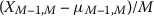is proved to converge in distribution to

$$\widetilde{Z}_{1} = \sum_{k=1}^{+\infty} \bigl(\widetilde{Y}_{k} - 1 / (p_{G}k) \bigr),$$

where $$\widetilde{Y}_{k}$$ are independent random variables with a exponential distribution with mean $$1/(p_{G}k)$$. $$\widetilde{Z}_{1}$$ has the same distribution as $$Z_{1}/p_{G}$$ in formula (21) in . Following the same steps as in , the convergence (6) is deduced.

To deduce (7) it is enough to use: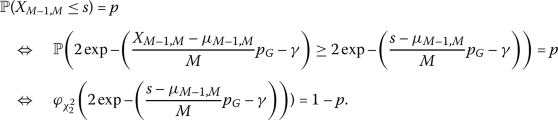□

### Proof

The proof is based on an asymptotic expansion of the characteristic function of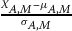,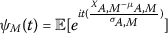.

By (3), we have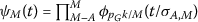, where $$\phi _{q}(t)$$ is the characteristic function of $$G_{q} - 1/q$$, with $$G_{q}$$ a Geometric distribution with parameter q.

Following the proof of Theorem 3, case (i) in , using an asymptotic expansion of $$\sigma _{A, M}$$ as $$M\to \infty$$, it is established that $$\psi _{M}(t) \to e^{-\frac {1}{2} t^{2}}$$, from which we deduce the asymptotic normality. □

### Proof

Consider the event $$X_{A, M} = s$$. The result of the sth shot corresponds therefore to a solution, it is the first occurrence of that solution and a total of $$A + 1$$ different solutions have appeared in the first s shots. To begin with, there are $${\binom{M}{A + 1}}$$ ways to choose those $$A + 1$$ solutions among the M possible solutions. We shall distinguish the cases depending on the number j of times, within the $$s-1$$ previous shots, that the output of Grover’s algorithm is not a solution. The integer j can take values between 0 and $$s - 1 - A$$, since at least A solutions have appeared.

In order to consider all possible events which union consists of $$X_{A, M} = s$$, consider a given value of j. We have to choose j positions among $$s - 1$$ possibilities for the ocurrences of “non solutions”. This amounts to $${\binom{s - 1}{j}}$$ possibilities. For the remaining $$s - 1 - j$$ shots, we must assign locations to each of the A different solutions. For that purpose, we first have to build a partition of the $$s - 1 - j$$ elements into A elements. There are $\left\{\begin{array}{c}s-1-j\\ A\end{array}\right\}$ ways to do so.

Moreover, the probability of such an individual event, where j shots of the algorithm do not output a solution, and $$s - j$$ shots correspond to a solution is:

$$\biggl({\frac {p_{G}}{M}} \biggr) ^{s - j} (1 - p_{G})^{j}.$$

Summing up, we obtain:

$${\mathbb{P}}(X_{A, M} = s) = {\binom{M}{A + 1}} (A + 1)! \sum _{j = 0} ^{s-1-A} {\binom{s-1}{j}} \begin{Bmatrix} s - 1- j \\ A \end{Bmatrix} \biggl({\frac {p_{G}}{M}} \biggr) ^{s - j}(1 - p_{G})^{j}.$$

Introducing the index $$l = s - j - 1$$ in the sum, the final formula (10) is deduced. □

## Availability of data and materials

Data sharing is not applicable to this article as no datasets were generated or analyzed during the current study. The repository https://github.com/mkesslerct/grover_shots contains an implementation of the formulae derived in this paper.

## References

1. Grover LK. A fast quantum mechanical algorithm for database search. In: Miller G, editor. Proceedings of the twenty-eighth annual ACM symposium on theory of computing. New York: ACM; 1996. p. 212–9.

2. Zalka C. Grover’s quantum searching algorithm is optimal. Phys Rev A. 1999;60:2746–51.

3. Boyer M, Brassard G, Høyer P, Tapp A. Tight bounds on quantum searching. Fortschr Phys. 1998;46:493–505.

4. Toyama FM, Van Dijk W, Nogami Y. Quantum search with certainty based on modified Grover algorithms: optimum choice of parameters. Quantum Inf Process. 2013;12:1897–914.

5. Long GL. Grover algorithm with zero theoretical failure rate. Phys Rev A. 2001;64:022307.

6. Grover LK. Quantum computers can search rapidly by using almost any transformation. Phys Rev Lett. 1998;80:4329–32.

7. Roy T, Jiang L, Schuster DI. Deterministic Grover search with a restricted oracle. Phys Rev Res. 2022;4:L022013.

8. Brassard G, Høyer P, Mosca M, Tapp A. Quantum amplitude amplification and estimation. In: Lomonaco S, Brandt H, editors. Proceedings of an AMS special session on quantum computation and information. 2002. p. 53–74.

9. Uno S, Suzuki Y, Hisanaga K, Raymond R, Tanaka T, Onodera T, Yamamoto N. Modified Grover operator for quantum amplitude estimation. New J Phys. 2021;23:083031.

10. Grinko D, Gacon J, Zoufal C, Woerner S. Iterative quantum amplitude estimation. npj Quantum Inf. 2021;7:52.

11. Tezuka H, Nakaji K, Satoh T, Yamamoto N. Grover search revisited: application to image pattern matching. Phys Rev A. 2022;105:032440.

12. Sakhi Z, Kabil R, Tragha A, Bennai M. Quantum cryptography based on Grover’s algorithm. In: 2nd international conference on innovative computing technology, INTECH 2012. 2012. p. 33–7.

13. Baritompa WP, Bulger DW, Wood GR. Grover’s quantum algorithm applied to global optimization. SIAM J Optim. 2005;15:1170–84.

14. Niroula P, Nam Y. A quantum algorithm for string matching. npj Quantum Inf. 2021;7:37.

15. Schmitz AT, Johri S. A quantum solution for efficient use of symmetries in the simulation of many-body systems. npj Quantum Inf. 2020;6:2.

16. Udrescu M, Prodan L, Vlǎduţiu M. Implementing quantum genetic algorithms: a solution based on Grover’s algorithm. In: Proceedings of the 3rd conference on computing frontiers 2006, CF ’06. vol. 2006. 2006. p. 71–81.

17. Acampora G, Luongo F, Vitiello A. Quantum implementation of fuzzy systems through Grover’s algorithm. In: IEEE international conference on fuzzy systems. 2018.

18. Alonso D, Sánchez P, Sánchez-Rubio F. Engineering the development of quantum programs: application to the Boolean satisfiability problem. Adv Eng Softw. 2022;173:103216.

19. Ristè D, da Silva MP, Ryan CA, Cross AW, Córcoles AD, Smolin JA, Gambetta JM, Chow JM, Johnson BR. Demonstration of quantum advantage in machine learning. npj Quantum Inf. 2017;3:16.

20. Grant E, Benedetti M, Cao S, Hallam A, Lockhart J, Stojevic V, Green AG, Severini S. Hierarchical quantum classifiers. npj Quantum Inf. 2018;4:65.

21. Isaac R. The pleasures of probability. New York: Springer; 1995.

22. Feller W. An introduction to probability theory and its applications. vol. 1. New Jersey: Wiley; 2009.

23. Brayton RK. On the asymptotic behavior of the number of trials necessary to complete a set with random selection. J Math Anal Appl. 1963;7:31–61.

24. Myers AN, Wilf HS. Some new aspects of the coupon collector’s problem. SIAM J Discrete Math. 2003;17:1–17.

25. Doumas AV, Papanicolaou VG. The coupon collector’s problem revisited: asymptotics of the variance. Adv Appl Probab. 2012;44:166–95.

26. Nielsen MA, Chuang IL. Quantum computation and quantum information. Cambridge: Cambridge University Press; 2000.

27. Egan L, Debroy DM, Noel C, Risinger A, Zhu D, Biswas D, Newman M, Li M, Brown KR, Cetina M, Monroe C. Fault-tolerant control of an error-corrected qubit. Nature. 2021;598(7880):281–6.

28. Salas PJ. Noise effect on Grover algorithm. Eur Phys J D. 2008;46:365–73.

29. Reitzner D, Hillery M. Grover search under localized dephasing. Phys Rev A. 2019;99:012339.

30. McNeil AJ, Frey R, Embrechts P. Quantitative risk management: concepts, techniques and tools. Princeton: Princeton University Press; 2015.

31. Baum LE, Billingsley P. Asymptotic distributions for the coupon collector’s problem. Ann Math Stat. 1965;36:1835–9.

32. NIST Digital Library of Mathematical Functions. http://dlmf.nist.gov/, Release 1.1.6 of 2022-06-30. F.W.J. Olver, A.B. Olde Daalhuis, D.W. Lozier, B.I. Schneider, R.F. Boisvert, C.W. Clark, B.R. Miller, B.V. Saunders, H.S. Cohl, and M.A. McClain, eds. http://dlmf.nist.gov/.

33. Wilf HS. Generating functionology. San Diego: Academic Press; 1994.

34. Granlund T, The GMP Development Team. GNU MP: the GNU multiple precision arithmetic library. vol. 5.0.5 edn. 2012. http://gmplib.org/.

35. Magnus R. Fundamental mathematical analysis. Cham: Springer; 2020.

36. Ross SM. A first course in probability. 8th ed. Upper Saddle River: Pearson Education; 2008.

## Funding

Mathieu Kessler acknowlegdes the support of Fundación Séneca-Agencia de Ciencia y Tecnología de la Región de Murcia (Grant 20911/PI/18).

## Author information

Authors

### Contributions

Mathieu Kessler: formal analysis, software, validation, visualization, writing-review. Pedro Sanchez and Diego Alonso: conceptualization, methodology, validation, investigation, writing-review.

### Corresponding author

Correspondence to Mathieu Kessler.

## Ethics declarations

### Competing interests

The authors declare no competing interests.

### Publisher’s Note

Springer Nature remains neutral with regard to jurisdictional claims in published maps and institutional affiliations.

## Appendix

### Appendix

For completion, the counterparts of Propositions 1, 2 and 3 for the original coupon collector problem are presented here.

The coupon collector problem: If each pack of bubble gum contains a coupon, and a complete set consists of M different coupons, how many packs of bubble gum must one buy to obtain a complete set with a probability of p? [21, Sect. 8.1 and 8.4].

Result 1. If T denotes the number of packs the collector has to buy before getting a complete set, the probability mass function of T is:

$$\text{For } s \in \mathbb{N}, s\geq M\text{:}\quad { \mathbb{P}}(T = s) = \sum_{i=1}^{M} { \binom{M}{i}} \biggl(\frac{M-i}{M} \biggr)^{s-1} \frac{i}{M} (-1)^{i+1}.$$
(12)

A proof of this result can be found in [36, p. 121].

It can be proved that formula (10) of Proposition 3 simplifies to (12) if one sets $$A = M -1$$ and $$p_{G} =1$$, which expresses the fact that the interest is in finding the M solution, i.e., $$T = X_{M-1, M}$$, and the probability to find a coupon in a pack is 1, respectively.

Regarding Propositions 1 and 2, their counterparts for the original coupon collector problem can be found as Theorem 4 and Theorem 3 in , respectively. The authors of this reference call $$W_{M}$$ the drawing, i.e., the pack, on which, for the first time the number of different coupons that have been obtained is $$A_{M}+ 1$$, and are interested in the asymptotic distribution of $$W_{M}$$ when M and $$A_{M}$$ both go to infinity. They derive different theorems depending on the rate of convergence of $$A_{M}$$ to infinity as $$M\to \infty$$. The two results relevant to Propositions 1, 2 are stated below for reference.

Result 2 (Theorem 4 in ). If $$M-A_{M}$$ is a constant b as M goes to infinity, then $$\exp \{-(W_{M}/M -\log 2M) \}$$ converges in law to the chi-square distribution with 2b degrees of freedom.

This result is the inspiration for Proposition 1, where $$A_{M} = M -1$$, which implies $$b = M-A_{M}=1$$ and expresses the fact the interest is in finding all M solutions.

Result 3 (Theorem 3 in ). If $$A_{M}/\sqrt{M}$$ and $$M-A_{M}$$ both go to infinity, then $$(W_{M} -\mathbb{E}[W_{M}])/\sqrt{\operatorname{var}(W_{M})}$$ converges in law to the normal distribution with mean 0 and variance 1.

This result is the inspiration for Proposition 2, where $$A_{M} = k\cdot M$$, for some $$0< k<1$$, which expresses the fact the interest is in finding a fraction of the M solutions.

## Rights and permissions